# Poisson Distribution¶

## If I throw 4000 marbles into 100 jars, how many jars will have exactly 42 marbles?¶

In :
import math
import random

num_marbles = 4000
num_jars    = 100

jars = []

## empty out all the jars

for j in xrange(num_jars):
jars.append(0)

## fill the jars randomly
for i in xrange(num_marbles):
jar = random.randint(0,num_jars-1) ## make sure in the range [0..num_jars-1]
jars[jar] += 1

## count how many jars have 42 marbles
target_val = 42
target_count = 0.

for j in xrange(num_jars):
if (jars[j] == target_val):
print "Jar %d has %d marbles" % (j, target_val)
target_count += 1

sample_mean = (sum(jars)+0.) / num_jars
print "There were %d jars with %d marbles for a probability of %.05f" % (target_count, target_val, target_count / num_jars)
print "The mean number of marbles per jar was %.02f" % (sample_mean)

Jar 23 has 42 marbles
Jar 37 has 42 marbles
Jar 43 has 42 marbles
Jar 48 has 42 marbles
Jar 74 has 42 marbles
Jar 80 has 42 marbles
There were 6 jars with 42 marbles for a probability of 0.06000
The mean number of marbles per jar was 40.00

In :
## Plot the number of marbles in each jar
import matplotlib.pyplot as plt
plt.figure(figsize=(15,4), dpi=100)
plt.xlabel("Jar Index")
plt.ylabel("Marbles in Jar")
plt.bar(range(num_jars), jars)
plt.show()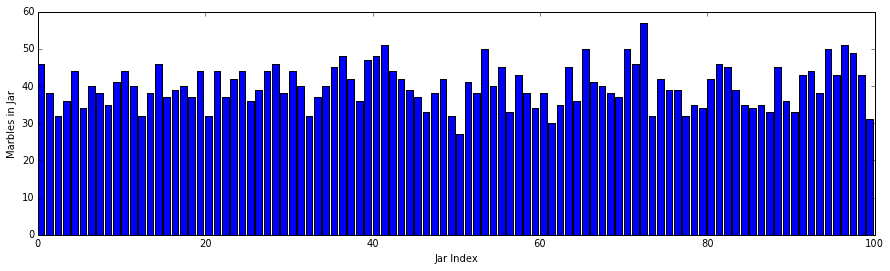In :
## Plot the distribution on the number of marbles in each jar
plt.figure()
plt.xlabel("Number of Marbles")
plt.ylabel("Number of Jars with that many marbles")
plt.hist(jars, bins=range(max(jars)+3))
plt.show()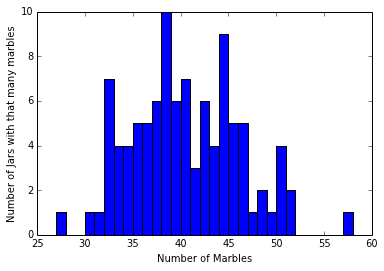In :
## Plot the density function, notice bars sum to exactly 1 but otherwise just scales display
plt.figure()
plt.xlabel("Number of Marbles")
plt.ylabel("Probability of Jars with that many marbles")
plt.hist(jars, bins=range(max(jars)+3), normed=True)
plt.show()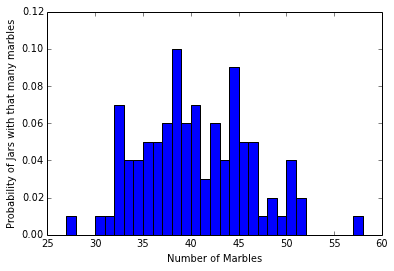In :
## Compute mean & stdev of distribution
mean = (sum(jars) + 0.) / num_jars
sumdiff = 0.0
for count in jars:
diff = (count - mean) ** 2
sumdiff += diff

sumdiff /= num_jars
stdev = math.sqrt(sumdiff)

print "The mean values was %0.2f +/- %0.2f" % (mean, stdev)

The mean values was 40.00 +/- 5.61


#### What happens to the mean or standard deviation if we change the number of jars or marbles?¶

In :
## Overlap the normal distribution with this mean and stdev
gaus_prob_dist = []
pi = 3.1415926

for k in xrange(int(max(jars)*1.2)+3):
var = float(stdev)**2
num = math.exp(-(float(k)-float(mean))**2/(2*var))
denom = (2*pi*var)**.5
gaus_prob = num/denom
gaus_prob_dist.append(gaus_prob)

## overlay the analytic distributions over the data
plt.figure()
plt.xlabel("Number of Marbles")
plt.ylabel("Probability of Jars with that many marbles")
plt.hist(jars, bins=range(int(max(jars)*1.2+3)), normed=True, label="Observed")
plt.plot(gaus_prob_dist, color='green', linewidth=4, linestyle='dashed', label="Normal Fit")
plt.legend(loc="upper left")
plt.show()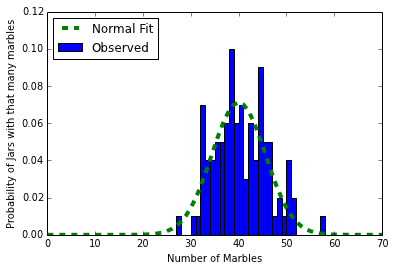In :
## Mean value is intuitively num_marbles / num_jars
## but what is the expected stdev?

expected_mean = num_marbles / num_jars
print "The range in values was %0.2f +/- %0.2f" % (mean, stdev)
print "The expected frequency is %.02f +/- ???" % (expected_mean)

The range in values was 40.00 +/- 5.61
The expected frequency is 40.00 +/- ???


#### Any guesses for stdev???¶

In :
## Lets simulate many experiments with different mean values to see what happens to the stdev
import sys
num_trials = 100
max_sim_mean = 1000

sim_target_means = []
sim_means  = []
sim_stdevs = []

for t in xrange(num_trials):
target_mean = random.randint(0,max_sim_mean)
num_marbles = num_jars * target_mean

sim_jars = []

## empty out all the jars

for j in xrange(num_jars):
sim_jars.append(0)

## fill the jars randomly
for i in xrange(num_marbles):
jar = random.randint(1,num_jars) - 1 ## make sure in the range 0..num_jars-1
sim_jars[jar] += 1

## Compute mean & stdev of distribution
sim_mean = (sum(sim_jars) + 0.) / num_jars
sumdiff = 0.0
for count in sim_jars:
diff = (count - sim_mean) ** 2
sumdiff += diff

sumdiff /= num_jars
sim_stdev = math.sqrt(sumdiff)

sim_target_means.append(target_mean)
sim_means.append(sim_mean)
sim_stdevs.append(sim_stdev)

if (t % 10 == 0):
print "Completed %d trials" % (t)


Completed 0 trials
Completed 10 trials
Completed 20 trials
Completed 30 trials
Completed 40 trials
Completed 50 trials
Completed 60 trials
Completed 70 trials
Completed 80 trials
Completed 90 trials

In :
## first make sure the target and simulated means agree
plt.figure()
plt.xlabel("Target means for simulations")
plt.ylabel("Simulated means")
plt.scatter(sim_target_means, sim_means)
plt.show()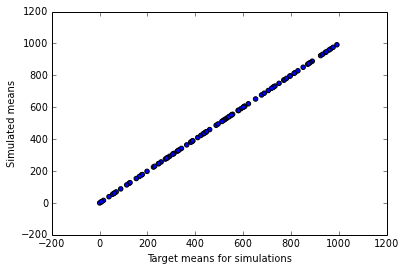In :
## Now plot the relationship between the mean and stdev of the different simulations
plt.figure()
plt.xlabel("Simulated means")
plt.ylabel("Simulated standard deviations")
plt.scatter(sim_means, sim_stdevs)
plt.show()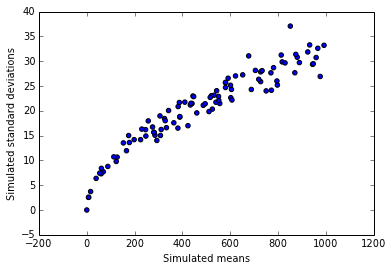In :
## Now plot the relationship between the mean and stdev of the different simulations
plt.figure()
plt.xlabel("Simulated means")
plt.ylabel("Simulated standard deviations")
plt.scatter(sim_means, sim_stdevs, label="observed")
sx = []
sy = []
for i in xrange(max_sim_mean):
sx.append(i)
sy.append(math.sqrt(i))
plt.plot(sx, sy, color='red', linewidth=4, label="sqrt")
plt.legend(loc="upper left")
plt.show()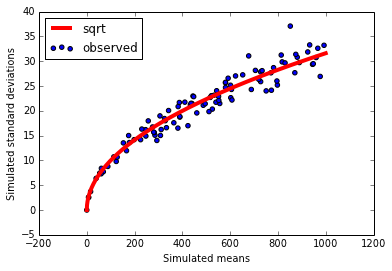Eureka! The data look normal and the stdev is consistently the sqrt of the mean!

This is the hallmark of the Poisson distribution

$$prob(\text{jar has k marbles given the mean amount}) = \frac{(mean^{k})}{k!} e^{-mean}$$
In :
prob_42 = math.exp(-sample_mean) * (sample_mean ** 42) / math.factorial(42)
print "The probability of having 42 marbles when the mean is %.02f is %.05f" % (sample_mean, prob_42)
print "There were %d jars with %d marbles for a probability of %.05f" % (target_count, target_val, target_count / num_jars)

The probability of having 42 marbles when the mean is 40.00 is 0.05849
There were 6 jars with 42 marbles for a probability of 0.06000

In :
## Compute the distribution analytically
pois_prob_dist = []

## This will crash for large sample means!
for k in xrange(int(max(jars)*1.2)+3):
prob_pois = math.exp(-sample_mean) * (sample_mean ** k) / math.factorial(k)
pois_prob_dist.append(prob_pois)

## Plot the poisson dist.
plt.figure()
plt.xlabel("Number of Marbles")
plt.ylabel("Probability of Jars with that many marbles")
plt.plot(pois_prob_dist, color='purple', linewidth=4, linestyle='solid')
plt.show()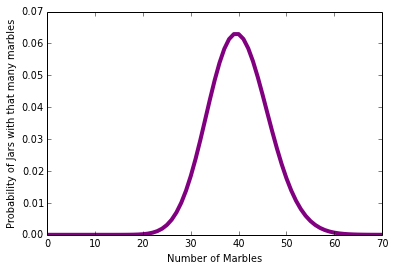In :
## overlay the analytic distributions over the data
plt.figure()
plt.xlabel("Number of Marbles")
plt.ylabel("Probability of Jars with that many marbles")
plt.hist(jars, bins=range(int(max(jars)*1.2)+3), normed=True, label="observed")
plt.plot(pois_prob_dist, color='purple', linewidth=4, linestyle='solid', label="Poisson Fit")
plt.plot(gaus_prob_dist, color='green', linewidth=4, linestyle='dashed', label="Normal Fit")
plt.legend(loc="upper left")
plt.show()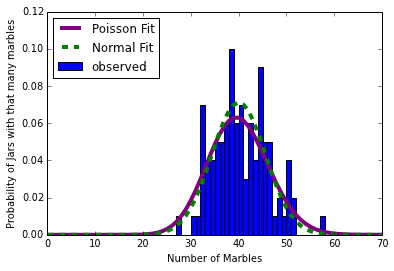## What happens for very small sample means?¶

In :